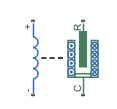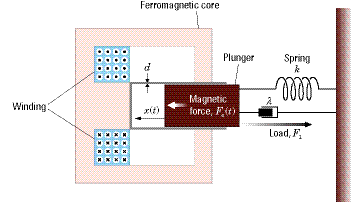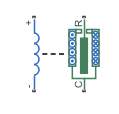# Solenoid

Electrical characteristics and generated force of solenoid

•Libraries:
Simscape / Electrical / Electromechanical / Mechatronic Actuators

## Description

The Solenoid block represents the electrical characteristics and generated force for the solenoid in this figure:Both positive and negative currents generate a force that pulls the plunger towards the winding. This minimizes the reluctance and decreases the stroke. To increase the stroke, a spring or an external force must overcome the magnetic force.

The return spring is optional. To remove the effects of this spring from the model, set the Spring constant parameter to `0`.

To show the direction in which the solenoid acts, the block icon changes dynamically based on value of the Motion direction under excitation parameter.

Note

If you place an external position sensor around the block, pay attention to the sensor orientation and the initial position condition. The initial position condition you specify on the sensor corresponds to the Stroke, X variable of the Solenoid block only if you orientate the sensor in a direction that is consistent with the value of the Motion direction under excitation parameter.

When The Motion direction under excitation Parameter Is Set To... Block Icon
`Port R moves away from port C``Port R moves towards port C`The equation of motion for the plunger as a function of position, x, is:

`${F}_{l}+m\stackrel{¨}{x}+\lambda \stackrel{˙}{x}+kx={F}_{e}$`

where Fe is the electromagnetic force, Fl is the load force, λ is the viscous damping term and m is the plunger mass. The electromagnetic force is related to the solenoid current and inductance by:

`${F}_{e}=\frac{1}{2}{i}^{2}\frac{\partial L\left(x\right)}{\partial x}$`

The inductance, which is derived in , can be written as:

`$\frac{\partial L\left(x\right)}{\partial x}=\frac{-\beta }{{\left(\alpha +\beta x\right)}^{2}}$`

where α and β are constants. Plugging the preceding equation into the equation for electromagnetic force gives the force-stroke relationship of the solenoid for a current i0:

`$F=\frac{1}{2}{i}_{0}{}^{2}\frac{-\beta }{{\left(\alpha +\beta x\right)}^{2}}$`

The Solenoid block solves for α and β by taking the two specified force and stroke measurements and substituting them into the preceding equation. It solves the resulting equations for α and β.

A positive current from the electrical + to - ports creates a negative force (i.e., a pulling force) from the mechanical C to R ports.

### Model Thermal Effects

You can expose the thermal port to model the effects of losses that convert power to heat. To expose the thermal port, set the Modeling option parameter to either:

• `No thermal port` — The block does not contain a thermal port.

• `Show thermal port` — The block contains one thermal conserving port.

For more information about using thermal ports in actuator blocks, see Simulating Thermal Effects in Rotational and Translational Actuators.

### Variables

To set the priority and initial target values for the block variables before simulation, use the Initial Targets section in the block dialog box or Property Inspector. For more information, see Set Priority and Initial Target for Block Variables.

Use nominal values to specify the expected magnitude of a variable in a model. Using system scaling based on nominal values increases the simulation robustness. Nominal values can come from different sources. One of these sources is the Nominal Values section in the block dialog box or Property Inspector. For more information, see System Scaling by Nominal Values.

Use the Stroke, X variable to set the target for the initial plunger position at the start of simulation.

Note

The Stroke, X variable is the initial air gap or stroke of the solenoid, and so should be greater than or equal to `0`.

## Ports

### Conserving

expand all

Electrical conserving port associated with the solenoid positive terminal.

Electrical conserving port associated with the solenoid negative terminal.

Mechanical translational conserving port associated with the case.

Mechanical translational conserving port associated with the plunger.

Thermal port.

#### Dependencies

To enable this port, set Modeling option to `Show thermal port`.

## Parameters

expand all

Whether to enable the thermal port of the block and model the effects of losses that convert power to heat.

### Magnetic Force

Select one of the following options for the type of motion direction under excitation:

• ```Port R moves away from port C```

• ```Port R moves towards port C```

A vector of the force values at the two points on the force-stroke curve. The second measurement point must be at a stroke that is greater than that of the first measurement point. When the manufacturer does not provide a force-stroke curve, set F1 to the holding force (when X1 = 0) and F2 to the pull-in force when running the solenoid at the Rated voltage Vdc and Rated current Idc values.

A vector of the stroke (plunger distance from the fully closed position) values at the two points on the force-stroke curve. The second measurement point must be at a stroke that is greater than that of the first measurement point. To ensure a finite force value, the points must meet the condition

`$\frac{X2}{X1}>\sqrt{\frac{F1}{F2}}$`

The voltage at which the solenoid is rated to operate. This voltage value is used to measure the Forces [F1 F2] and Stroke [X1 X2] values.

The current that flows when the solenoid is supplied with the Rated voltage Vdc voltage.

### Mechanical

Constant representing the stiffness of the spring that acts to retract the plunger when the solenoid is powered off. The force is zero when the plunger is displaced to the Stroke for zero spring force parameter value. Set the spring constant to zero if there is no spring.

The stroke at which the spring provides no force.

The term λ in the equation of motion for the plunger as a function of position that linearly damps the plunger motion. The value can be zero.

The weight of the solenoid plunger. The value can be zero.

The maximum amount by which the plunger can be displaced. You can use this parameter to model a hard endstop that limits the stroke. The default value is `Inf` mm, which means no stroke limit.

Stiffness of the plunger contact that models the hard stop at the minimum (x = 0) and maximum (x = Maximum stroke) plunger positions.

Damping of the plunger contact that models the hard stop at the minimum (x = 0) and maximum (x = Maximum stroke) plunger positions.

### Thermal

To enable these parameters, set Modeling option to `Show thermal port`.

Resistance temperature coefficient.

Temperature for which the actuator parameters are defined.

Thermal mass is the energy required to raise the temperature by one degree.

 S.E. Lyshevski. Electromechanical Systems, Electric Machines, and Applied Mechatronics. CRC, 1999.